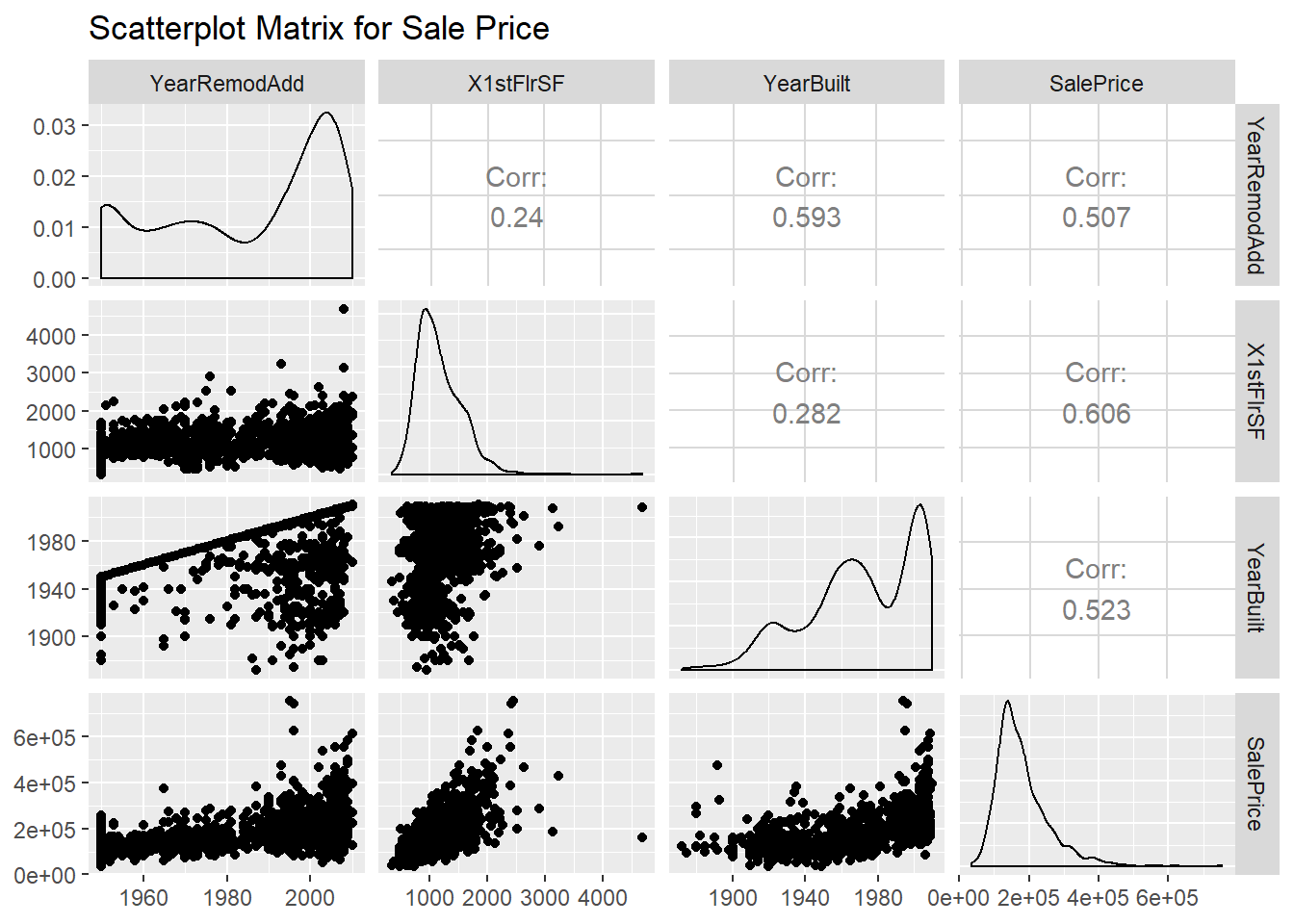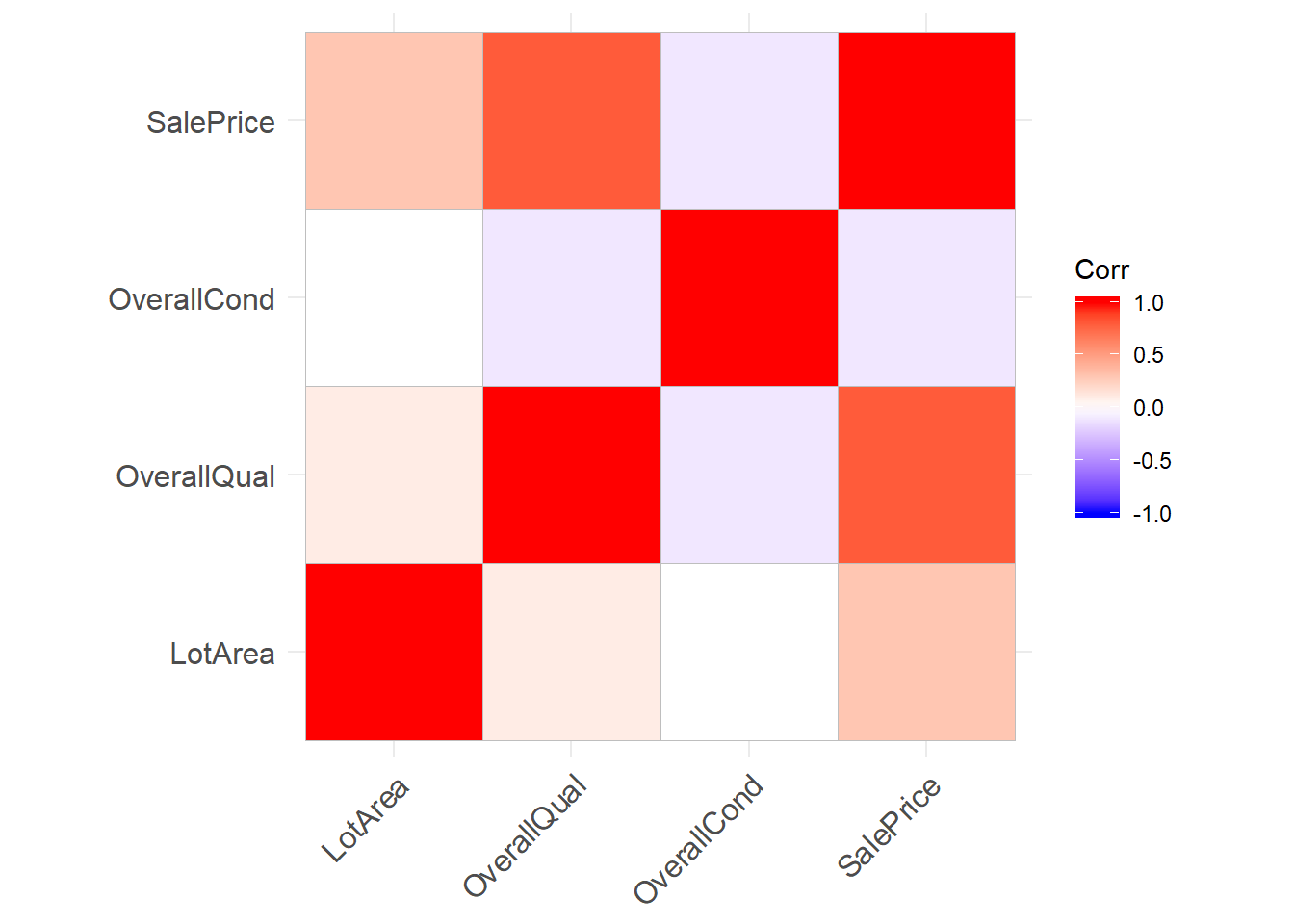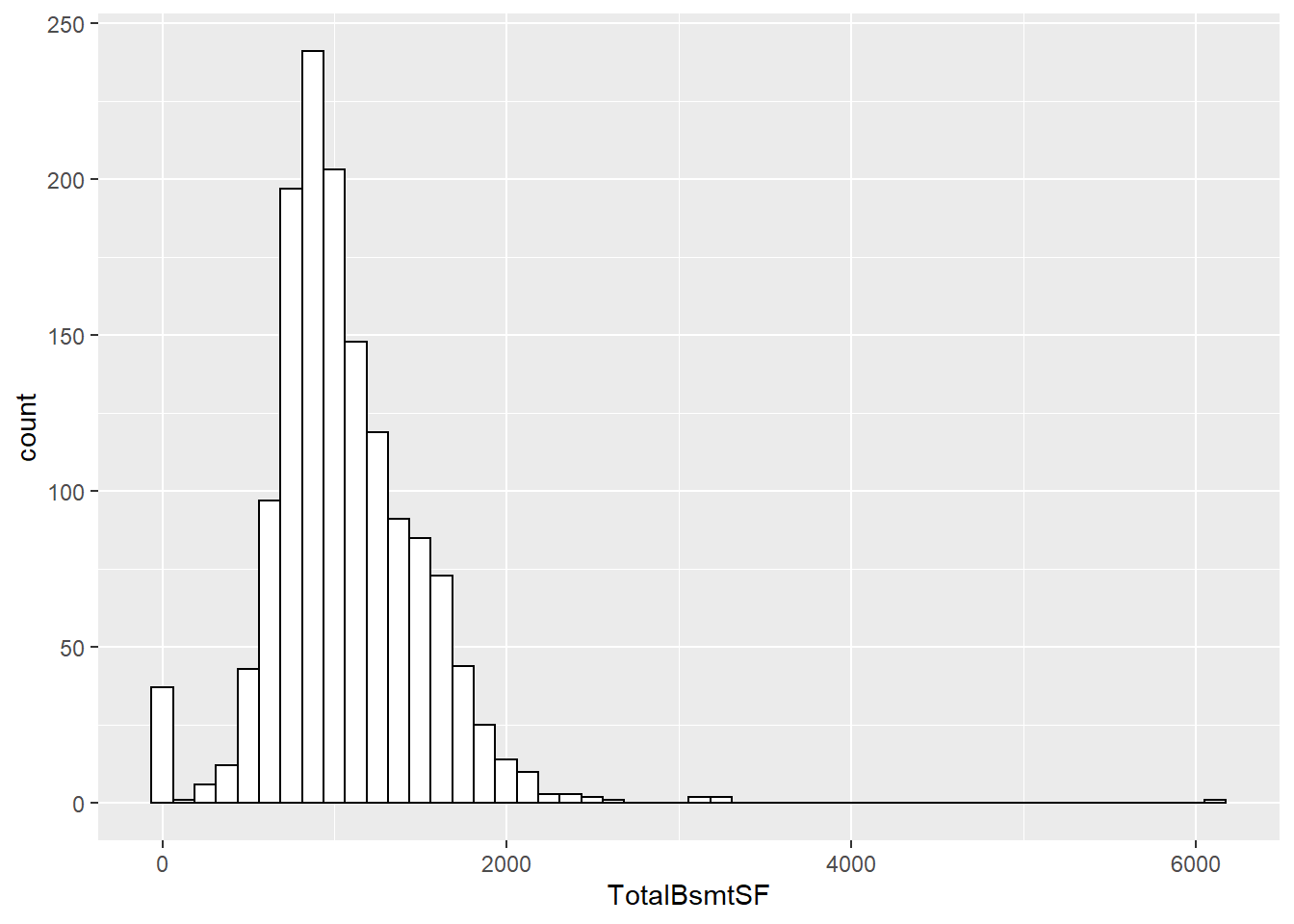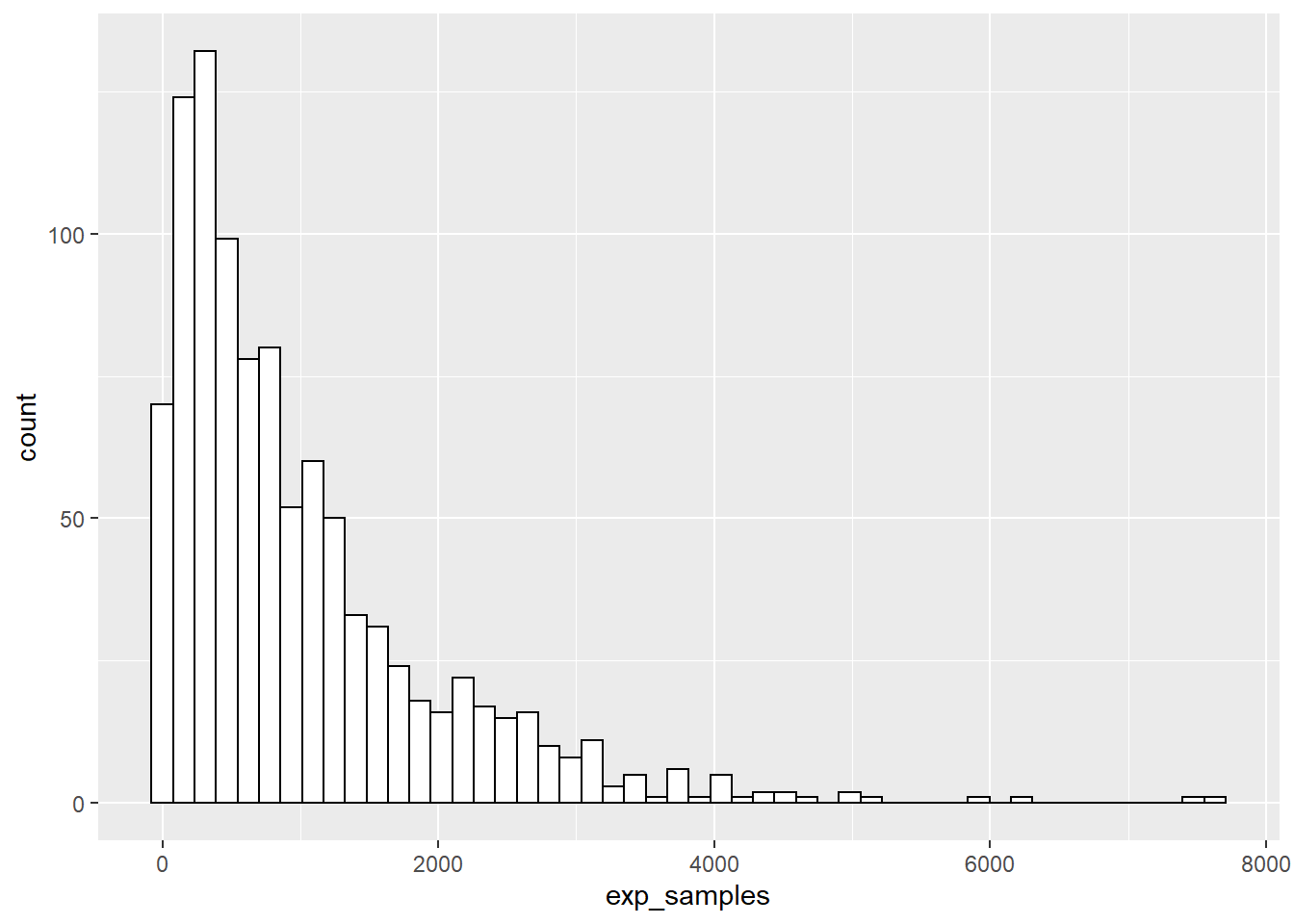## Problem 1

### Random Variables

Using R, generate a random variable X that has 10,000 random uniform numbers from 1 to N, where N can be any number of your choosing greater than or equal to 6.

##generate a random variable X that has 10,000 random uniform numbers from 1 to N, where N can be any number of your choosing greater than or equal to 6
rand_var = function(n) runif(10000,1, n)

##set n equal to 6
n = 6

X <- rand_var(n)
summary(X)
##    Min. 1st Qu.  Median    Mean 3rd Qu.    Max.
##   1.000   2.239   3.529   3.519   4.794   6.000

Then generate a random variable Y that has 10,000 random normal numbers with a mean of: $\mu = \sigma = \frac{(N+1)}{2}$

#generate a random variable Y that has 10,000 random normal numbers with a mean and sd of mu
mu = (n + 1)/2
Y <- rnorm(10000,mean=mu, sd = mu)
summary(Y)
##    Min. 1st Qu.  Median    Mean 3rd Qu.    Max.
##  -9.482   1.143   3.515   3.490   5.882  16.238

### Probability

5 points. Calculate as a minimum the below probabilities a through c. Assume the small letter “x” is estimated as the median of the X variable, and the small letter “y” is estimated as the 1st quartile of the Y variable. Interpret the meaning of all probabilities.

#median of X variable
x <- quantile(X)[]
print(x)
##  3.529372
#1st quartile of Y variable
y <- quantile(Y)[]
print(y)
##  1.143492

#### P(X>x | X>y)

This is the probability that X is greater than the median of X given that X is greater than the first quartile of Y.

Since the random variables are independent, the conditional probability will be:

$P(X>x | X>y) = \frac{P(X>x, X>y)}{P(X>y)} = \frac{P(X>x) \times P(X>y)}{P(X>y)} = P(X>x)$

P(X>x) = 0.5 P(X>x | X>y) = 0.5

Experimental

mean(X>x)*mean(X>y)/mean(X>y)
##  0.5

#### P(X>x, Y>y)

This is the probability that X is greater than its median and Y is greater than its first quartile Since the median of the variable is its midpoint, P(X>x) = 0.5. Similarly, since y is the first quartile, P(Y>y) = 0.75.

$P(X>x, Y>y) = P(X>x) \times P(Y>y) = 0.5 \times 0.75 = 0.375$

Experimental

mean(X>x)*mean(Y>y)
##  0.375

#### (X<x | X>y)

This is the probability that X is less than its median given that it is greater than the first quartile of y.

$P(X<x | X>y) = \frac{P(X<x, X>y)}{P(X>y)} = \frac{P(X<x) \times P(X>y)}{P(X>y)} = P(X<x) = 0.5$

This example probability is the inverse of example a, so it will be equal to 1 - P(X>x|X>y) = 0.5

Experimental

mean(X<x)*mean(X>y)/mean(X>y)
##  0.5

### Marginal / Joint

5 points.
Investigate whether P(X>x and Y>y)=P(X>x)P(Y>y) by building a table and evaluating the marginal and joint probabilities.

Marginal Probabilities: The probability of a single event.

• P(X>x)
• P(X<x)
• P(Y>y)
• P(Y<y)
marginal <- cbind(cbind(mean(X>x), mean(X<x)),cbind(mean(Y>y), mean(Y<y)))
colnames(marginal) <- c('P(X>x)','P(X<x)','P(Y>y)','P(Y<y)')
#rownames(marginal) <- c('P(Y>y)','P(Y<y)')
marginal
##      P(X>x) P(X<x) P(Y>y) P(Y<y)
## [1,]    0.5    0.5   0.75   0.25

Joint probabilities: The probability of two events occuring simultaneously.

• P(X>x and Y>y)
• P(Xy)
• P(X>x and Y<y)
• P(X<x and Y<y)
joint <- rbind(cbind(mean(X>x)*mean(Y>y), mean(X<x)*mean(Y>y)),cbind(mean(Y<y)*mean(X>x), mean(Y<y)*mean(X<x)))
colnames(joint) <- c('P(X>x)','P(X<x)')
rownames(joint) <- c('P(Y>y)','P(Y<y)')
joint
##        P(X>x) P(X<x)
## P(Y>y)  0.375  0.375
## P(Y<y)  0.125  0.125

### Independence

5 points.
Check to see if independence holds by using Fisher’s Exact Test and the Chi Square Test. What is the difference between the two? Which is most appropriate?

Fisher’s Exact Test

Tests independence of rows and columns in a contingency table with fixed marginal probabilities. According to the test, since the p-value = 1, we fail to reject the null hypothesis that rows and columns are independent.

# fisher test - multiplied by 10000 (amount of trials) to remove error 'x' has been rounded to integer: Mean relative difference: 1
fisher.test(joint*10000, alternative = "two.sided", conf.int = FALSE, conf.level = 0.95)
##
##  Fisher's Exact Test for Count Data
##
## data:  joint * 10000
## p-value = 1
## alternative hypothesis: true odds ratio is not equal to 1
## sample estimates:
## odds ratio
##          1

Chi Square Test

Tests independence of rows and columns - whether the probability distributions of X and Y are not affected by each other. According to the test, since the p-value = 1, we fail to reject the null hypothesis that rows and columns are independent.

library(MASS)
## Warning: package 'MASS' was built under R version 3.6.1
# chisq test - multiplied by 10000 (amount of trials) to remove error
chisq.test(joint*10000) 
##
##  Pearson's Chi-squared test
##
## data:  joint * 10000
## X-squared = 0, df = 1, p-value = 1

Conclusion

The difference between the Chi-squared test and Fisher’s exact test is that the latter is more accurate while the former is an approximation. However, in this situation, since we have 10,000 random variables, the Chi-square test is more appropriate; the Chi-square test is better for large sample sizes.

## Problem 2

You are to register for Kaggle.com (free) and compete in the House Prices: Advanced Regression Techniques competition. https://www.kaggle.com/c/house-prices-advanced-regression-techniques

#get datasets
train <- read.csv(file = "https://raw.githubusercontent.com/mkivenson/Computational-Mathematics/master/Final%20Project/train.csv")

### Descriptive and Inferential Statistics

#### Univariate Descriptive Statistics

Provide univariate descriptive statistics and appropriate plots for the training data set.

The following provides descriptive statistics for each column in the training data set. Further data visualization can be found below and in the model portion of the project.

summary(train$SalePrice) ## Min. 1st Qu. Median Mean 3rd Qu. Max. ## 34900 129975 163000 180921 214000 755000 #### Scatterplot Matrix Provide a scatterplot matrix for at least two of the independent variables and the dependent variable. library(ggplot2) colnames(train) ##  "Id" "MSSubClass" "MSZoning" "LotFrontage" ##  "LotArea" "Street" "Alley" "LotShape" ##  "LandContour" "Utilities" "LotConfig" "LandSlope" ##  "Neighborhood" "Condition1" "Condition2" "BldgType" ##  "HouseStyle" "OverallQual" "OverallCond" "YearBuilt" ##  "YearRemodAdd" "RoofStyle" "RoofMatl" "Exterior1st" ##  "Exterior2nd" "MasVnrType" "MasVnrArea" "ExterQual" ##  "ExterCond" "Foundation" "BsmtQual" "BsmtCond" ##  "BsmtExposure" "BsmtFinType1" "BsmtFinSF1" "BsmtFinType2" ##  "BsmtFinSF2" "BsmtUnfSF" "TotalBsmtSF" "Heating" ##  "HeatingQC" "CentralAir" "Electrical" "X1stFlrSF" ##  "X2ndFlrSF" "LowQualFinSF" "GrLivArea" "BsmtFullBath" ##  "BsmtHalfBath" "FullBath" "HalfBath" "BedroomAbvGr" ##  "KitchenAbvGr" "KitchenQual" "TotRmsAbvGrd" "Functional" ##  "Fireplaces" "FireplaceQu" "GarageType" "GarageYrBlt" ##  "GarageFinish" "GarageCars" "GarageArea" "GarageQual" ##  "GarageCond" "PavedDrive" "WoodDeckSF" "OpenPorchSF" ##  "EnclosedPorch" "X3SsnPorch" "ScreenPorch" "PoolArea" ##  "PoolQC" "Fence" "MiscFeature" "MiscVal" ##  "MoSold" "YrSold" "SaleType" "SaleCondition" ##  "SalePrice" library(ggplot2) library(GGally) ## Warning: package 'GGally' was built under R version 3.6.1 ## Registered S3 method overwritten by 'GGally': ## method from ## +.gg ggplot2 ggpairs(train, columns=c(21,44,20,81), aes()) + ggtitle("Scatterplot Matrix for Sale Price")#### Correlation Matrix Derive a correlation matrix for any three quantitative variables in the dataset. This correlation matrix will show OverallQual, OverallCond, LotArea, and SalePrice. library(ggcorrplot) ## Warning: package 'ggcorrplot' was built under R version 3.6.1 corr <- round(cor(train[, c(5,18,19,81)]), 1) ggcorrplot(corr)#### Hypothesis Test the hypotheses that the correlations between each pairwise set of variables is 0 and provide an 80% confidence interval. Discuss the meaning of your analysis. Would you be worried about familywise error? Why or why not? #LotArea and OverallQual c1 <- cor.test(x = corr[1,], y = corr[2,], method = "pearson", conf.level = 0.8) print(paste("P-value:", c1$p.value, " | ", "Conf Int:", c1$conf.int, "-", c1$conf.int))
##  "P-value: 0.736136559675603  |  Conf Int: -0.914083312120158 - 0.766297160828907"
#LotArea and OverallCond
c2 <- cor.test(x = corr[1,], y = corr[3,], method = "pearson", conf.level = 0.8)
print(paste("P-value:", c2$p.value, " | ", "Conf Int:", c2$conf.int, "-", c2$conf.int)) ##  "P-value: 0.558189701264645 | Conf Int: -0.942057888073233 - 0.667972762518936" #LotArea and SalePrice c3 <- cor.test(x = corr[1,], y = corr[4,], method = "pearson", conf.level = 0.8) print(paste("P-value:", c3$p.value, " | ", "Conf Int:", c3$conf.int, "-", c3$conf.int))
##  "P-value: 0.97023203007135  |  Conf Int: -0.864611132667099 - 0.84878057107682"
#OverallQual and OverallCond
c4 <- cor.test(x = corr[2,], y = corr[3,], method = "pearson", conf.level = 0.8)
print(paste("P-value:", c4$p.value, " | ", "Conf Int:", c4$conf.int, "-", c4$conf.int)) ##  "P-value: 0.251449188515929 | Conf Int: -0.978078315801338 - 0.302165269266376" #OverallQual and SalePrice c5 <- cor.test(x = corr[2,], y = corr[4,], method = "pearson", conf.level = 0.8) print(paste("P-value:", c5$p.value, " | ", "Conf Int:", c5$conf.int, "-", c5$conf.int))
##  "P-value: 0.0669468207577246  |  Conf Int: 0.379886616460239 - 0.994676257665829"
#OverallCond and SalePrice
c6 <- cor.test(x = corr[3,], y = corr[4,], method = "pearson", conf.level = 0.8)
print(paste("P-value:", c6$p.value, " | ", "Conf Int:", c6$conf.int, "-", c6$conf.int)) ##  "P-value: 0.14759924204137 | Conf Int: -0.987793842434317 - 0.016681514522772" The correlation tests show that the correlations between OverallQual & SalePrice is significant, since the confidence interval does not include a correlation of 0. Familywise error, or Type I error, is the probability of making a false conclusion. We should be worried about familywise error - the probability of it occuring is equal to the significance value (0.20) ### Linear Algebra and Correlation #### Precision Matrix Invert your correlation matrix from above. (This is known as the precision matrix and contains variance inflation factors on the diagonal.) I used the built-in inv() function in R to invert the correlation matrix. library(matlib) ## Warning: package 'matlib' was built under R version 3.6.1 precision <- inv(corr) precision ## ## [1,] 1.16951380 0.45335085 -0.02628121 -0.71616294 ## [2,] 0.45335085 2.95663601 0.04599212 -2.49671485 ## [3,] -0.02628121 0.04599212 1.01182654 0.07227332 ## [4,] -0.71616294 -2.49671485 0.07227332 3.21944809 Multiply the correlation matrix by the precision matrix, and then multiply the precision matrix by the correlation matrix. The product of these matrix operations is the identity matrix. round(precision %*% corr,2) ## LotArea OverallQual OverallCond SalePrice ## [1,] 1 0 0 0 ## [2,] 0 1 0 0 ## [3,] 0 0 1 0 ## [4,] 0 0 0 1 round(corr %*% precision) ## ## LotArea 1 0 0 0 ## OverallQual 0 1 0 0 ## OverallCond 0 0 1 0 ## SalePrice 0 0 0 1 #### LU Decomposition Conduct LU decomposition on the matrix. I used the function that I created in a previous homework assignment to perform LU decomposition. lu <- function(matrix){ n = nrow(matrix) lower = matrix((c(1:n)*0), nrow=n, ncol=n) upper = matrix((c(1:n)*0), nrow=n, ncol=n) for(i in (1:n)) { for(k in (i:n)) { sum = 0 for(j in (1:i)) { sum = sum + (lower[i,j] * upper[j, k]) } upper[i, k] = matrix[i, k] - sum } for(k in (i:n)) { if(i == k) { lower[i, i] = 1 } else { sum = 0 for(j in (1:i)) { sum = sum + (lower[k, j] * upper[j, i]) } lower[k, i] = (matrix[k, i] - sum) / upper[i, i] } } } return(list(lower, upper)) } lu(corr) ## [] ## [,1] [,2] [,3] [,4] ## [1,] 1.0 0.0000000 0.00000000 0 ## [2,] 0.1 1.0000000 0.00000000 0 ## [3,] 0.0 -0.1010101 1.00000000 0 ## [4,] 0.3 0.7777778 -0.02244898 1 ## ## [] ## [,1] [,2] [,3] [,4] ## [1,] 1 0.10 0.000000 0.30000000 ## [2,] 0 0.99 -0.100000 0.77000000 ## [3,] 0 0.00 0.989899 -0.02222222 ## [4,] 0 0.00 0.000000 0.31061224 lu(precision) ## [] ## [,1] [,2] [,3] [,4] ## [1,] 1.00000000 0.00000000 0.0 0 ## [2,] 0.38764045 1.00000000 0.0 0 ## [3,] -0.02247191 0.02020202 1.0 0 ## [4,] -0.61235955 -0.79797980 0.1 1 ## ## [] ## [,1] [,2] [,3] [,4] ## [1,] 1.169514 0.4533509 -0.02628121 -0.7161629 ## [2,] 0.000000 2.7808989 0.05617978 -2.2191011 ## [3,] 0.000000 0.0000000 1.01010101 0.1010101 ## [4,] 0.000000 0.0000000 0.00000000 1.0000000 ### Calculus-Based Probability & Statistics #### Shift Distribution Many times, it makes sense to fit a closed form distribution to data. Select a variable in the Kaggle.com training dataset that is skewed to the right, shift it so that the minimum value is absolutely above zero if necessary. TotalBsmtSF is skewed to the right since its mean is greater than its median. All its values are already above zero. # Draw with black outline, white fill ggplot(train, aes(x=TotalBsmtSF)) + geom_histogram(bins=50, colour="black", fill="white")summary(train$TotalBsmtSF)
##    Min. 1st Qu.  Median    Mean 3rd Qu.    Max.
##     0.0   795.8   991.5  1057.4  1298.2  6110.0

#### Exponential Probability Density

Then load the MASS package and run fitdistr to fit an exponential probability density function. (See https://stat.ethz.ch/R-manual/R-devel/library/MASS/html/fitdistr.html ). Find the optimal value of lambda for this distribution, and then take 1000 samples from this exponential distribution using this value (e.g., rexp(1000, lambda)).

lambda <- fitdistr(train$TotalBsmtSF, "exponential")$estimate[]
lambda
##  0.0009456896
exp_samples <- rexp(1000, lambda)

#### Plot Histogram

Plot a histogram and compare it with a histogram of your original variable. Using the exponential pdf, find the 5th and 95th percentiles using the cumulative distribution function (CDF). Also generate a 95% confidence interval from the empirical data, assuming normality. Finally, provide the empirical 5th percentile and 95th percentile of the data. Discuss.

This new histogram has the shape of an exponential distribution, while the original variable, though skewed, is closer to a normal distribution.

ggplot(as.data.frame(exp_samples), aes(x=exp_samples)) +
geom_histogram(bins=50, colour="black", fill="white")95% Confidence

library(EnvStats)
## Warning: package 'EnvStats' was built under R version 3.6.1
##
## Attaching package: 'EnvStats'
## The following object is masked from 'package:MASS':
##
##     boxcox
## The following objects are masked from 'package:stats':
##
##     predict, predict.lm
## The following object is masked from 'package:base':
##
##     print.default
eexp(train$TotalBsmtSF, ci = TRUE, conf.level = 0.95) ## ## Results of Distribution Parameter Estimation ## -------------------------------------------- ## ## Assumed Distribution: Exponential ## ## Estimated Parameter(s): rate = 0.0009456896 ## ## Estimation Method: mle/mme ## ## Data: train$TotalBsmtSF
##
## Sample Size:                     1460
##
## Confidence Interval for:         rate
##
## Confidence Interval Method:      Exact
##
## Confidence Interval Type:        two-sided
##
## Confidence Level:                95%
##
## Confidence Interval:             LCL = 0.0008977972
##                                  UCL = 0.0009948089

CDF vs Emperical percentials

#CDF
quantile(exp_samples, c(.05, .95)) 
##         5%        95%
##   60.57627 2921.96747
#Emperical
quantile(train\$TotalBsmtSF, c(.05, .95)) 
##     5%    95%
##  519.3 1753.0

## Modeling

Build some type of multiple regression model and submit your model to the competition board. Provide your complete model summary and results with analysis. Report your Kaggle.com user name and score.

Kaggle Account: mkivenson

Score: 0.13507

Link to regression model: Regression Model Notebook